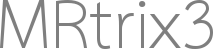# Non-default connectome contrasts

The `tck2connectome` command will by default produce a connectome where the value stored in each edge corresponds to the number of streamlines ascribed to that particular pair of nodes. If individual streamline weights (as e.g. calculated by the `tcksift2` command) are also provided via the `-tck_weights_in` option, then the value stored in each edge becomes the sum of the weights of the streamlines ascribed to each particular edge.

These are however not the only contrasts (i.e. quantitative property stored in each edge of the network) that this command is capable of providing. Examples of some of the more common alternative usages are provided below.

(Note that these contrasts are independent of the `-tck_weights_in` option: all of the mechanisms described below can be used either with or without additionally providing streamline weights)

### “Mean FA” / “Mean (quantitative_image_contrast)”

This approach involves taking some quantitative image metric (e.g. Fractional Anisotropy from the diffusion tensor model), and for each connectome edge, calculating the mean of that quantitative metric within those voxels occupied by the streamlines corresponding to that edge.

As far as I can tell, this is typically achieved using the following approach:

• Extract the streamlines corresponding to a particular connectome edge
• Generate a voxel map of streamline count
• Apply some threshold to produce a voxel mask
• Calculate the mean of the quantitative metric within that mask
• Repeat for all connectome edges

While this approach is certainly possible using MRtrix3 commands, it’s not the approach adopted in `tck2connectome`. Instead, the mean of the quantitative metric of interest along each streamline is calculated; then, for a particular connectome edge, the mean of these values across those streamlines ascribed to that edge is calculated. Apart from the computational advantages, this also means that the contribution of different voxels toward the eventual value obtained for any particular connectome edge is weighted according to the frequency with which different voxels are traversed by the streamlines ascribed to that edge. Contrast this with the approach described in dot point form above, where a voxel at the outer extremity of the mask contributes toward the mean just as much as a voxel right in the middle of the pathway.

``````\$ tcksample tracks.tck scalar.mif mean_scalars.csv -stat_tck mean
\$ tck2connectome tracks.tck nodes.mif connectome.csv -scale_file mean_scalars.csv -stat_edge mean
``````

The first step samples the image `scalar.mif` along each streamline, calculates the mean sampled value along each streamline , and stores these values into file `mean_scalars.csv` (one value for every streamline). The second step then assigns the value associated with each streamline to be assigned to the relevant edge during connectome construction to be the corresponding values from this file, and finally calculates the value of each edge to be the mean of the values across the streamlines in that edge. So there are actually two “mean” operations happening here: One for the mean value along each streamline, and one for the mean of the values across streamlines within each connectome edge.

### Mean streamline length

``````\$ tck2connectome tracks.tck nodes.mif connectome.csv -scale_length -stat_edge mean
``````

For each streamline, the contribution of that streamline to the relevant edge is scaled by the length of that streamline; so, in the absence of any other scaling, the contribution of that streamline will be equal to the length of the streamline in mm. Finally, for each edge, take the mean of the values contributed from all streamlines belonging to that edge. This, by construction, is in fact the “mean” “length”.### Scaling by inverse streamline length / inverse node volumes

``````\$ tck2connectome tracks.tck nodes.mif connectome.csv -scale_invlength -scale_invnodevol
``````

The `-scale_invlength` and `-scale_invnodevol` options provide easy access to the ability to scale the contribution of each streamline by the reciprocal of its length, and scale the value stored in each connectome edge by the reciprocals of the volumes of the two relevant nodes, respectively, as described in Patric Hagmann’s seminal 2008 paper. Do not however interpret the presence of these options as advocating their use.